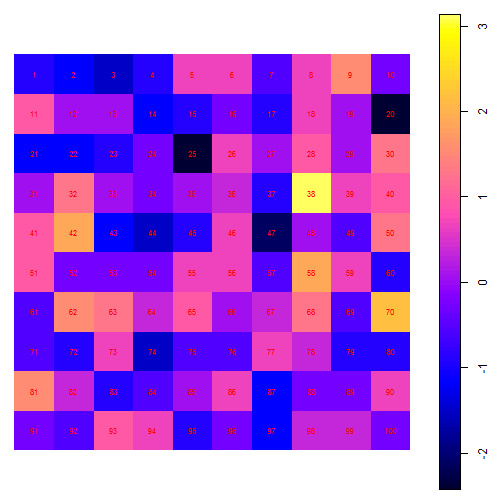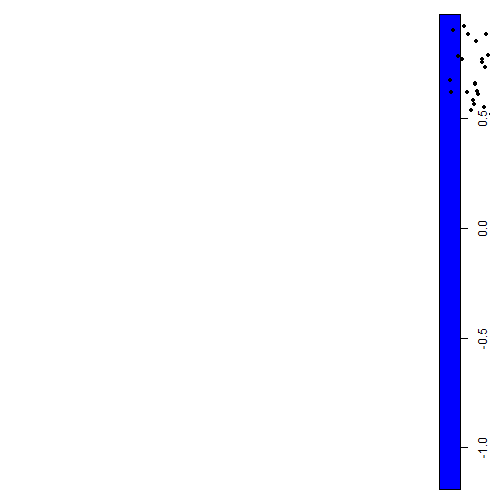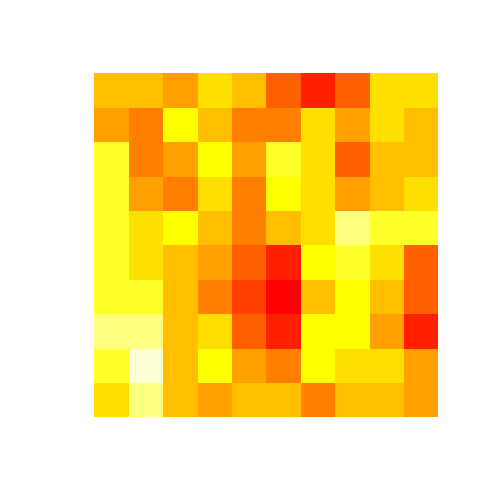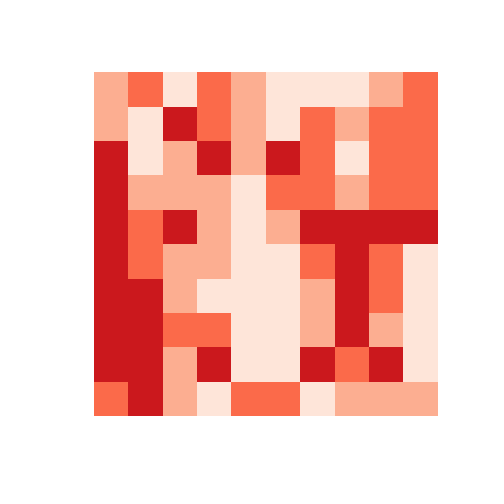# Hierarchical Spatial Simultaneous Autoregressive Model (HSAR)

A detailed synthetic example will illustrate the use of the hsar() function, including the generation of attribute data,spatial data and the extraction of the spatial weights matrices and the random effect design matrix.

### Libraries

In order to run the example, sp, maptools, rdgal and rgeos libraries will be loaded.

``````library(maptools)
library(rgdal)
library(rgeos)
``````

### Creating synthetic data

Initialy we set up a grid data representing higher-level units, say neighbourhoods

``````grid_high <- GridTopology(cellcentre.offset=c(1,1),cellsize=c(1,1),cells.dim=c(10,10))
grid_sp <- SpatialGrid(grid_high)
``````

then we create an attribute, say population, for the grid cells

``````population <- as.numeric(scale(rpois(100,10)))
``````

and create a SpatialGridDataFrame

``````grid_data <- SpatialGridDataFrame(grid_sp,data.frame(population))
``````

Now check the names of each grid cell and plot the four cells from the left-top corner. Also, let's create lower-level units, says 2000 individuals

``````plot(grid_data)
text(coordinates(grid_data),row.names(grid_data),col="red",cex=0.7)
````````````plot(grid_data[1:2,1:2,"population"],add=TRUE,col="blue",lwd=3)

individual_points <- spsample(grid_data,2000,"random")
row.names(individual_points) <- as.character(1:2000)
points(individual_points,pch=16,cex=0.7)
``````and create some attributes for individuals

``````att.data <- data.frame(happiness=as.numeric(scale(runif(2000,1,10))),
income=as.numeric(scale(runif(2000,1,10))),
age=as.numeric(scale(round(runif(2000,20,50)))))
row.names(att.data) <- as.character(1:2000)
individual_data <- SpatialPointsDataFrame(individual_points,att.data)
``````

### Create matrices used in the hsar function

Now we extract the random effect design matrix and two spatial weights matrices to use the hsar() function.

So we start by finding out the neighbouhood where each individual is located based on the spatial locations

``````link.index <- over(individual_points,grid_sp)
``````

and by extracting the population attribute of the neighbourhood where an individual is located

``````link.data <- over(individual_data,grid_data)
``````

Next we order the data so that the neighbourhood index is ordered ascendingly

``````model.data.sp <- individual_data[order(individual_data\$neighbourhood_index),]
``````

and we can have a look at the first 10 observations

``````head(model.data.sp@data,10)
``````
``````##        happiness     income        age neighbourhood_index population
## 344   0.92403639  0.9552658  0.3437325                   1 -0.8806948
## 508  -1.18371161  0.6745939 -1.2842291                   1 -0.8806948
## 585   1.34942887  0.6332669  1.1577132                   1 -0.8806948
## 687   0.02024352  0.5314872 -0.3539654                   1 -0.8806948
## 789   1.11028380 -1.1066955 -1.6330780                   1 -0.8806948
## 839  -1.50313934 -0.3521266  1.5065621                   1 -0.8806948
## 847  -1.18974128 -0.6344352  1.0414303                   1 -0.8806948
## 853   0.55578797 -1.2006361  1.7391281                   1 -0.8806948
## 982   0.30767385 -1.0453654  0.4600154                   1 -0.8806948
## 1315 -1.00330033  1.7101341 -0.9353802                   1 -0.8806948
``````

Let's define the random effect matrix which captures the number of individuals within each neighbourhood

``````MM <- as.data.frame(table(model.data.sp\$neighbourhood_index))
``````

Find the total number of neighbourhood (100 in our case)

``````Utotal <- dim(MM)
Unum <- MM[,2]
Uid <- rep(c(1:Utotal),Unum)
``````

Define the random effect matrix (Delta) with a dimension of 2000x100

``````library(spdep)
n <- nrow(model.data.sp)
Delta <- matrix(0,nrow=n,ncol=Utotal)
for(i in 1:Utotal) {
Delta[Uid==i,i] <- 1
}
rm(i)

Delta <- as(Delta,"dgCMatrix")
``````

Evaluate the neighbourhood-level (higher-level) spatial weights matrix based on sharing a common border (Rook's rule). Here we should note that if neighbourhoods are represented by spatial polygons, the extraction of the neighbour list and weights matrix is obtained through the poly2nb() and nb2listw() (or nb2mat()) functions in the R spdep package.

``````temp.coords <- coordinates(grid_sp)
distance <- array(0,c(Utotal,Utotal))
M <- array(0,c(Utotal,Utotal))
for(i in 1:Utotal) {
for(j in 1:Utotal){
temp <- (temp.coords[i,1] - temp.coords[j,1])^2 + (temp.coords[i,2] - temp.coords[j,2])^2
distance[i,j] <- sqrt(temp)
if(temp == 1) M[i,j] <- 1
}
}
``````

Then row-normalize M

``````M <- M / rowSums(M)
M <- as(M,"dgCMatrix")
``````

Then create the individual-level spatial weights matrix W. We simply assume each individual is interacting with other individuals located within a radius of 1.5 units. The intensity of interaction is measured by how far individuals are separated with a Gaussian distance decay kernel

``````library(RColorBrewer)
library(classInt)
``````

So create the neighbour list

``````nb.15 <- dnearneigh(model.data.sp,0,1.5)
``````

and the weights matrix W

``````dist.15 <- nbdists(nb.15,model.data.sp)
dist.15 <- lapply(dist.15,function(x) exp(-0.5 * (x / 1.5)^2))
mat.15 <- nb2mat(nb.15,glist=dist.15,style="W")
W <- as(mat.15,"dgCMatrix")
``````

### Run the models

Now let's simulate our outcome variable i.e. health of each individual using arbitary values of model parameters.

``````rho <- 0.2
lambda <- 0.7
sigma2e <- 0.8
sigma2u <- 0.4
betas <- c(3,2,3,4,5)
``````

In order to run th emodels we simulate neighbourhood-level random effect

``````thetas <- as.numeric(solve(diag(Utotal) - lambda*M) %*% rnorm(Utotal,mean=0,sd=sqrt(sigma2u)))
grid_data\$thetas_true <- thetas
image(grid_data,"thetas_true")
``````and simulate an individual-level outcome variable — health

``````X.mat <- as.matrix(model.data.sp@data[,c("happiness","income","age","population")])
X.mat <- cbind(rep(1,n),X.mat)
health <- as.numeric(solve(diag(n) - rho*W) %*% (X.mat %*% betas + Delta %*% thetas + rnorm(n,0,sqrt(sigma2e))))
model.data.sp\$health <- health
``````

So we wun the hsar() function

``````library(HSAR)
res.formula <- health ~ happiness + income + age + population
res <- hsar(res.formula,data=model.data.sp@data,W=W,M=M,Delta=Delta,burnin=100, Nsim=200)
summary(res)
``````
``````##
## Call:
## hsar(formula = res.formula, data = model.data.sp@data, W = W,
##     M = M, Delta = Delta, burnin = 100, Nsim = 200)
## Type:  hsar
##
## Coefficients:
##   (Intercept) happiness   income      age population
## 1    2.799396  1.949272 2.999727 3.967149   5.052837
##
##  Spatial Coefficients:
##          rho  lambda
## [1,] 0.19099 0.76155
##
##  Diagnostics
## Deviance information criterion (DIC): 5357.995
## Effective number of parameters (pd): 130.1882
## Log likelihood: -2548.809
## Pseudo R squared: 0.985815
##
##  Impacts:
##               direct  indirect    total
## (Intercept) 2.800391 0.6597150 3.460106
## happiness   1.949964 0.4593718 2.409336
## income      3.000793 0.7069256 3.707718
## age         3.968559 0.9349115 4.903470
## population  5.054632 1.1907683 6.245400
``````

and visualise the estimated neighbourhood effect

``````grid_data\$theta.est <- thetas.est <- as.numeric(res\$Mus)
image(grid_data,"theta.est",breaks=as.numeric(quantile(thetas.est)),col=brewer.pal(4,"Reds"))
``````Furher let's run another two simpler models Firstly, a model assuming rho = 0, that is, no interaction effects at the individual level

``````res_1 <- hsar(res.formula,data=model.data.sp@data,W=NULL,M=M,Delta=Delta,burnin=100, Nsim=200)
summary(res_1)
``````

and secondly, a model assuming lambda = 0, that is, no interaction effects at the neighbourhood level

``````res_2 <- hsar(res.formula,data=model.data.sp@data,W=W,M=NULL,Delta=Delta,burnin=100, Nsim=200)
summary(res_2)
``````